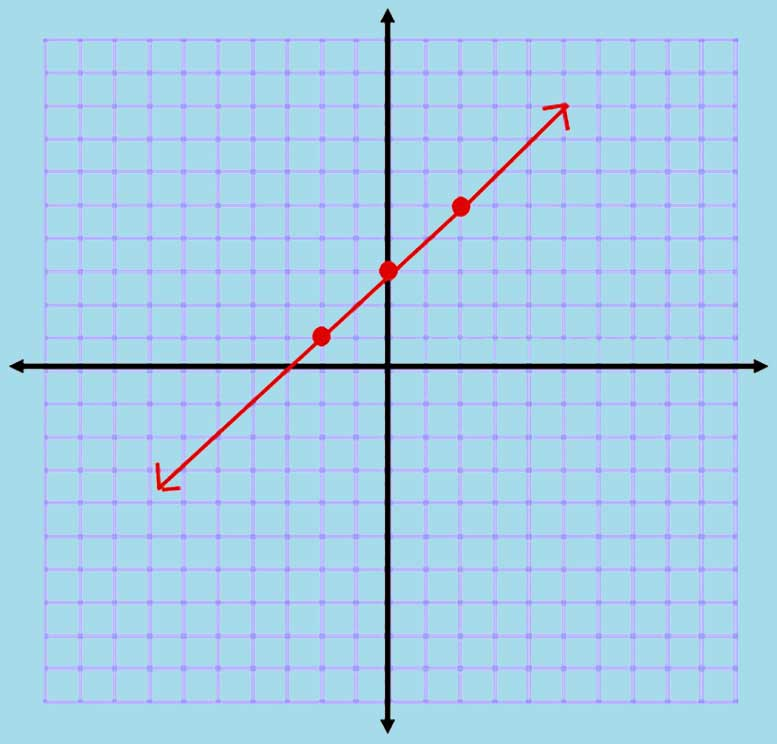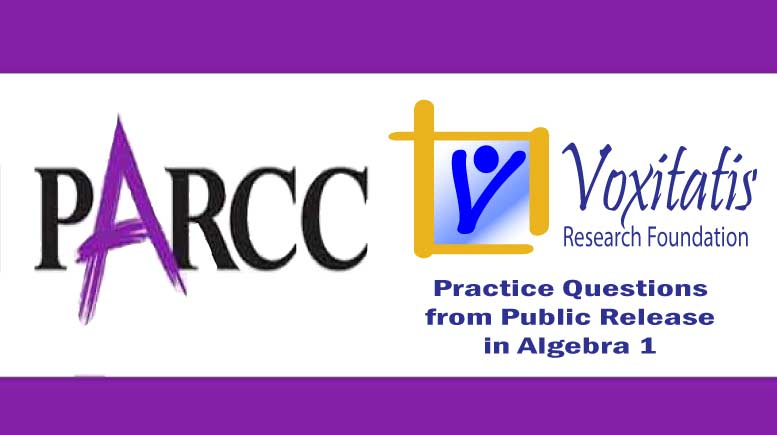Sunday, April 18, 2021# Algebra 1 PARCC question: proof of slope

-

#### The following multi-part constructed response question, explained here in hopes of helping algebra students in Maryland and Illinois prepare for the PARCC test near the end of this school year, appears on the released version of PARCC’s Spring 2015 test in algebra 1, here:Slope of a line given points on the line (blamevaraia / Flickr Creative Commons)

### Part A

Suppose that y = 2x – 3. The following points lie on the graph of this equation.

• A (a, 2a–3)
• B (b, 2b–3)
• C (c, 2c–3)

Amy claims that the slopes of$\overline{AB} \textrm{,}\enskip\overline{BC}\textrm{, and}\enskip\overline{AC}$ are equal. Prove that Amy’s claim is correct. Show your work and explain your reasoning.

Enter your answer, your work, and your explanation in the space provided.

### Part B

Are the points (–1, 1) and (1, –1) on the graph of y = 2x – 3?

Show your work and explain your reasoning.

Enter your answer, your work, and your explanation in the space provided.

Answer and references

Correct answers: Problem says Amy is correct, proof is human-scored. Use reasoning and show calculations.

PARCC evidence statement(s) tested: HS.C.18.1:

Construct, autonomously, chains of reasoning that will justify or refute propositions or conjectures about linear equations in one or two variables.

Content scope: 8.EE.B (For both Algebra1 and Math 1, we are revisiting content initially introduced in grade 8, from a more mature reasoning perspective).

The evidence statement above references Math Practice 3 (construct viable arguments and critique the reasoning of others) and Math Practice 6 (attend to precision) in the Common Core:

[MP.3] Mathematically proficient students understand and use stated assumptions, definitions, and previously established results in constructing arguments. They make conjectures and build a logical progression of statements to explore the truth of their conjectures. They are able to analyze situations by breaking them into cases, and can recognize and use counterexamples. They justify their conclusions, communicate them to others, and respond to the arguments of others. They reason inductively about data, making plausible arguments that take into account the context from which the data arose. Mathematically proficient students are also able to compare the effectiveness of two plausible arguments, distinguish correct logic or reasoning from that which is flawed, and—if there is a flaw in an argument—explain what it is. … Students learn to determine domains to which an argument applies. Students at all grades can listen or read the arguments of others, decide whether they make sense, and ask useful questions to clarify or improve the arguments.

The question requires students to understand content in the Common Core eighth-grade standard 8.EE.B.5, found under expressions and equations, which states that they should be able to “graph proportional relationships, interpreting the unit rate as the slope of the graph. Compare two different proportional relationships represented in different ways. For example, compare a distance-time graph to a distance-time equation to determine which of two moving objects has greater speed.”

Example of a solution strategy (there are others)

Line between any two points has a slope of 2. Plug in x and find y as the other member of the two ordered pairs.

For Part A, we have to prove that the slope of those three line segments is the same. It’s logical that it would be, since we are given that all three points lie on the same line, given by the equation. The equation itself isn’t really important, but if you are told that three points lie on the same line, then the slope between any two of those points must be the same. The slope of a line is constant along the entire line, from negative infinity to positive infinity.

We can do some calculations as well to check this reasoning. The general formula for the slope of a line, given two points on the line, as we have here, is as follows:$m = \frac{y_1 - y_0}{x_1 - x_0}$

Between A and B, assuming they don’t refer to the same point, we have a slope of$\frac{(2b-3)-(2a-3)}{b-a} = \frac{2b-3-2a+3}{b-a} = \frac{2b-2a}{b-a} = \frac{2(b-a)}{(b-a)} = 2$

Between B and C, assuming they don’t refer to the same point, we have a slope of$\frac{(2c-3)-(2b-3)}{c-b} = \frac{2c-3-2b+3}{c-b} = \frac{2c-2b}{c-b} = \frac{2(c-b)}{(c-b)} = 2$

Between A and C, assuming they don’t refer to the same point, we have a slope of$\frac{(2c-3)-(2a-3)}{c-a} = \frac{2c-3-2a+3}{c-a} = \frac{2c-2a}{c-a} = \frac{2(c-a)}{(c-a)} = 2$

And in Part B, it’s a simple matter of plugging the x value in each ordered pair into the equation for the line and finding y, which will or won’t be the y value given.

For (–1, 1):$y = 2(-1)-3 = -2-3 = -5\quad\textrm{not on the graph}$

For (1, –1):$y = 2(1)-3 = 2-3 = -1\quad\textrm{yes, on the graph}$

## Resources for further study

Purple Math, developed by Elizabeth Stapel, a math teacher from the St Louis area, has a few pages on determining the slope of a line and uses some of the same types of equations I did above. The pages start here.

The Khan Academy, developed by Sal Khan, an engineer who has created a library of thousands of video lessons, has several videos dealing with the determination of the slope of a line, including this one that describes how to determine the slope of a line given two points.

Appendix B-3 of Paul A Foerster’s book Algebra and Trigonometry deals with reasoning by mathematical induction, as required to solve this problem. The root principle of mathematical induction is this:

If you can show that:

1. Assuming that one statement in a series of statements being true means that the next statement is true, and
2. One of the statements in the series of statements actually is true,

then you can conclude that all of the statements are true, starting with the one that actually is true and moving forward.

Complete reference: Foerster, Paul A. Algebra and Trigonometry: Functions and Applications, revised edition. Addison-Wesley, 1980, 1984. The book is used in several algebra classes taught in middle and high schools in both Illinois and Maryland.

## Analysis of this question and online accessibility

The question measures knowledge of the Common Core standard and math practices it purports to measure and tests students’ ability to make reasonable conclusions about a line based on their knowledge of lines and the properties of lines. It is considered to have a median cognitive demand.

The question can be tested online and should yield results that are as valid and reliable as those obtained on paper. Students online may experience difficulties using the equation editor to show their math in determining the slope. We have previously described problems with the user interface of the equation editor tool (see here), and we will not repeat that argument here. However, we will use the opportunity to remind those of you taking the PARCC math test to spend a little extra time on problems with the equation editor to:

• Make sure you have typed in all the work necessary to make your case
• Transfer all your work from scratch paper to the computer so you can receive credit for it

No special accommodation challenges can be identified with this question, so the question is considered fair.

## ChallengeProve the distributive property by induction: a(x1 + … + xn) = ax1 + … + axn

You will need to incorporate the well-ordering axiom, which says that in any non-empty set of positive integers, there exists a least element.

## Purpose of this series of posts

Voxitatis is developing blog posts that address every algebra 1 question released to the public by the Partnership for Assessment of Readiness for College and Careers, or PARCC, in order to help students prepare to take the test this spring.

Our total release will run from February 27 through March 15, with one or two questions discussed per day. Then we’ll move to geometry at the end of March, algebra 2 during the first half of April, and eighth grade during the last half of April.Paul Katulahttps://news.schoolsdo.org
Paul Katula is the executive editor of the Voxitatis Research Foundation, which publishes this blog. For more information, see the About page.

### Md. to administer tests in math, English

0
Students in Md. will still have to take standardized tests this spring in math and English language arts, following action of the state board.